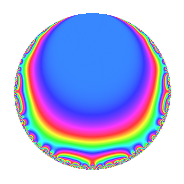# Properties

 Label 630.2.k.fLevel 630 Weight 2 Character orbit 630.k Analytic conductor 5.031 Analytic rank 0 Dimension 2 CM No Inner twists 2

# Related objects

## Newspace parameters

 Level: $$N$$ = $$630 = 2 \cdot 3^{2} \cdot 5 \cdot 7$$ Weight: $$k$$ = $$2$$ Character orbit: $$[\chi]$$ = 630.k (of order $$3$$ and degree $$2$$)

## Newform invariants

 Self dual: No Analytic conductor: $$5.03057532734$$ Analytic rank: $$0$$ Dimension: $$2$$ Coefficient field: $$\Q(\sqrt{-3})$$ Coefficient ring: $$\Z[a_1, a_2]$$ Coefficient ring index: $$1$$ Sato-Tate group: $\mathrm{SU}(2)[C_{3}]$

## $q$-expansion

Coefficients of the $$q$$-expansion are expressed in terms of a primitive root of unity $$\zeta_{6}$$. We also show the integral $$q$$-expansion of the trace form.

 $$f(q)$$ $$=$$ $$q + \zeta_{6} q^{2} + ( -1 + \zeta_{6} ) q^{4} -\zeta_{6} q^{5} + ( -1 + 3 \zeta_{6} ) q^{7} - q^{8} +O(q^{10})$$ $$q + \zeta_{6} q^{2} + ( -1 + \zeta_{6} ) q^{4} -\zeta_{6} q^{5} + ( -1 + 3 \zeta_{6} ) q^{7} - q^{8} + ( 1 - \zeta_{6} ) q^{10} + ( -2 + 2 \zeta_{6} ) q^{11} + ( -3 + 2 \zeta_{6} ) q^{14} -\zeta_{6} q^{16} + ( -4 + 4 \zeta_{6} ) q^{17} + 6 \zeta_{6} q^{19} + q^{20} -2 q^{22} + 3 \zeta_{6} q^{23} + ( -1 + \zeta_{6} ) q^{25} + ( -2 - \zeta_{6} ) q^{28} -9 q^{29} + ( 4 - 4 \zeta_{6} ) q^{31} + ( 1 - \zeta_{6} ) q^{32} -4 q^{34} + ( 3 - 2 \zeta_{6} ) q^{35} + 4 \zeta_{6} q^{37} + ( -6 + 6 \zeta_{6} ) q^{38} + \zeta_{6} q^{40} + 7 q^{41} -5 q^{43} -2 \zeta_{6} q^{44} + ( -3 + 3 \zeta_{6} ) q^{46} + 8 \zeta_{6} q^{47} + ( -8 + 3 \zeta_{6} ) q^{49} - q^{50} + ( -2 + 2 \zeta_{6} ) q^{53} + 2 q^{55} + ( 1 - 3 \zeta_{6} ) q^{56} -9 \zeta_{6} q^{58} + ( 10 - 10 \zeta_{6} ) q^{59} -\zeta_{6} q^{61} + 4 q^{62} + q^{64} + ( 9 - 9 \zeta_{6} ) q^{67} -4 \zeta_{6} q^{68} + ( 2 + \zeta_{6} ) q^{70} -2 q^{71} + ( 4 - 4 \zeta_{6} ) q^{73} + ( -4 + 4 \zeta_{6} ) q^{74} -6 q^{76} + ( -4 - 2 \zeta_{6} ) q^{77} -10 \zeta_{6} q^{79} + ( -1 + \zeta_{6} ) q^{80} + 7 \zeta_{6} q^{82} + 7 q^{83} + 4 q^{85} -5 \zeta_{6} q^{86} + ( 2 - 2 \zeta_{6} ) q^{88} + \zeta_{6} q^{89} -3 q^{92} + ( -8 + 8 \zeta_{6} ) q^{94} + ( 6 - 6 \zeta_{6} ) q^{95} + 14 q^{97} + ( -3 - 5 \zeta_{6} ) q^{98} +O(q^{100})$$ $$\operatorname{Tr}(f)(q)$$ $$=$$ $$2q + q^{2} - q^{4} - q^{5} + q^{7} - 2q^{8} + O(q^{10})$$ $$2q + q^{2} - q^{4} - q^{5} + q^{7} - 2q^{8} + q^{10} - 2q^{11} - 4q^{14} - q^{16} - 4q^{17} + 6q^{19} + 2q^{20} - 4q^{22} + 3q^{23} - q^{25} - 5q^{28} - 18q^{29} + 4q^{31} + q^{32} - 8q^{34} + 4q^{35} + 4q^{37} - 6q^{38} + q^{40} + 14q^{41} - 10q^{43} - 2q^{44} - 3q^{46} + 8q^{47} - 13q^{49} - 2q^{50} - 2q^{53} + 4q^{55} - q^{56} - 9q^{58} + 10q^{59} - q^{61} + 8q^{62} + 2q^{64} + 9q^{67} - 4q^{68} + 5q^{70} - 4q^{71} + 4q^{73} - 4q^{74} - 12q^{76} - 10q^{77} - 10q^{79} - q^{80} + 7q^{82} + 14q^{83} + 8q^{85} - 5q^{86} + 2q^{88} + q^{89} - 6q^{92} - 8q^{94} + 6q^{95} + 28q^{97} - 11q^{98} + O(q^{100})$$

## Character Values

We give the values of $$\chi$$ on generators for $$\left(\mathbb{Z}/630\mathbb{Z}\right)^\times$$.

 $$n$$ $$127$$ $$281$$ $$451$$ $$\chi(n)$$ $$1$$ $$1$$ $$-\zeta_{6}$$

## Embeddings

For each embedding $$\iota_m$$ of the coefficient field, the values $$\iota_m(a_n)$$ are shown below.

For more information on an embedded modular form you can click on its label.

Label $$\iota_m(\nu)$$ $$a_{2}$$ $$a_{3}$$ $$a_{4}$$ $$a_{5}$$ $$a_{6}$$ $$a_{7}$$ $$a_{8}$$ $$a_{9}$$ $$a_{10}$$
361.1
 0.5 + 0.866025i 0.5 − 0.866025i
0.500000 + 0.866025i 0 −0.500000 + 0.866025i −0.500000 0.866025i 0 0.500000 + 2.59808i −1.00000 0 0.500000 0.866025i
541.1 0.500000 0.866025i 0 −0.500000 0.866025i −0.500000 + 0.866025i 0 0.500000 2.59808i −1.00000 0 0.500000 + 0.866025i
 $$n$$: e.g. 2-40 or 990-1000 Significant digits: Format: Complex embeddings Normalized embeddings Satake parameters Satake angles

## Inner twists

Char. orbit Parity Mult. Self Twist Proved
1.a Even 1 trivial yes
7.c Even 1 yes

## Hecke kernels

This newform can be constructed as the intersection of the kernels of the following linear operators acting on $$S_{2}^{\mathrm{new}}(630, [\chi])$$:

 $$T_{11}^{2} + 2 T_{11} + 4$$ $$T_{13}$$ $$T_{17}^{2} + 4 T_{17} + 16$$